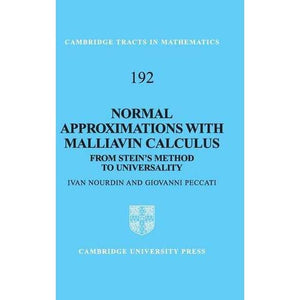># Normal Approximations with Malliavin Calculus: From Stein's Method to Universality (Cambridge Tracts in Mathematics)

• £33.49
• Save £29

Giovanni Peccati Ivan Nourdin
Cambridge University Press, 5/10/2012
EAN 9781107017771, ISBN10: 1107017777

Hardcover, 254 pages, 22.9 x 15.2 x 1.8 cm
Language: English

Stein's method is a collection of probabilistic techniques that allow one to assess the distance between two probability distributions by means of differential operators. In 2007, the authors discovered that one can combine Stein's method with the powerful Malliavin calculus of variations, in order to deduce quantitative central limit theorems involving functionals of general Gaussian fields. This book provides an ideal introduction both to Stein's method and Malliavin calculus, from the standpoint of normal approximations on a Gaussian space. Many recent developments and applications are studied in detail, for instance: fourth moment theorems on the Wiener chaos, density estimates, BreuerÃ¢â‚¬â€œMajor theorems for fractional processes, recursive cumulant computations, optimal rates and universality results for homogeneous sums. Largely self-contained, the book is perfect for self-study. It will appeal to researchers and graduate students in probability and statistics, especially those who wish to understand the connections between Stein's method and Malliavin calculus.

Preface
Introduction
1. Malliavin operators in the one-dimensional case
2. Malliavin operators and isonormal Gaussian processes
3. Stein's method for one-dimensional normal approximations
4. Multidimensional Stein's method
5. Stein meets Malliavin
univariate normal approximations
6. Multivariate normal approximations
7. Exploring the BreuerÃ¢â‚¬â€œMajor Theorem
8. Computation of cumulants
9. Exact asymptotics and optimal rates
10. Density estimates
11. Homogeneous sums and universality
Appendix 1. Gaussian elements, cumulants and Edgeworth expansions
Appendix 2. Hilbert space notation
Appendix 3. Distances between probability measures
Appendix 4. Fractional Brownian motion
Appendix 5. Some results from functional analysis
References
Index.